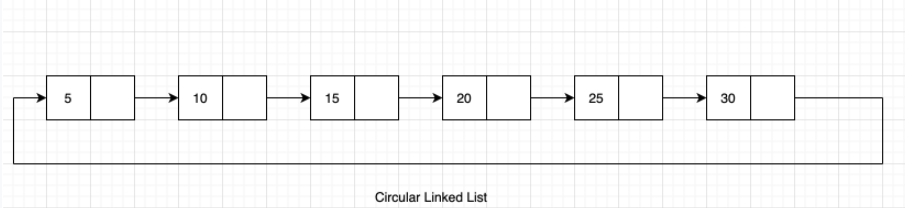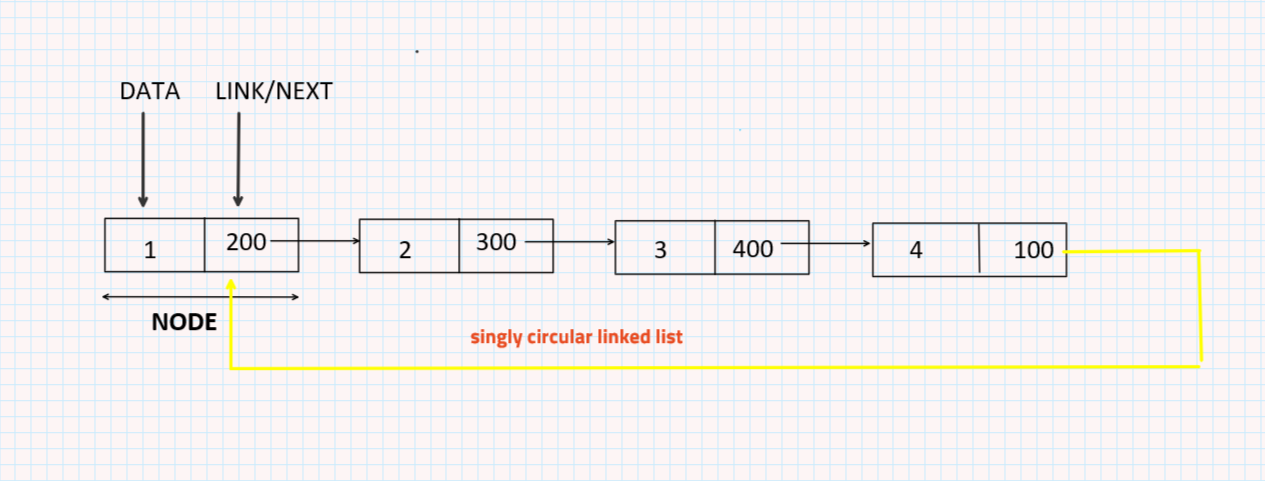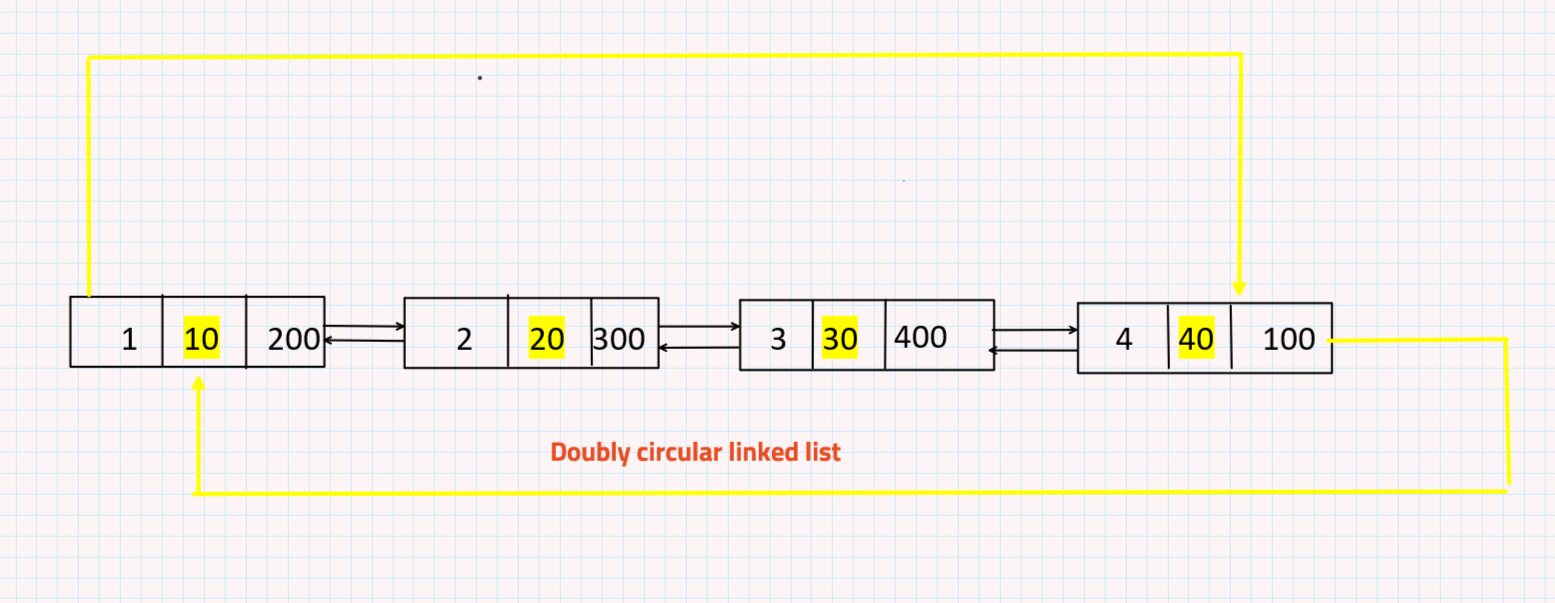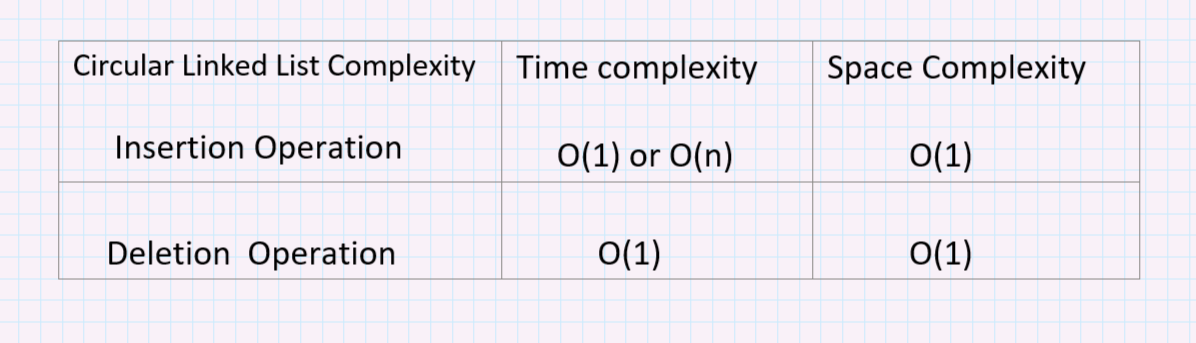# Circular Linked List in JavaCircular Linked List is a type of Linked List where the first and last nodes are connected to form a circle. In singly linked lists and doubly linked lists, the end of lists is indicated with a NULL value. But circular linked lists do not have ends i.e no node with a NULL pointer.

### Applications of Circular Linked List :

• Round Robin Algorithm is the best use case of Circular Linked List.
• Circular linked lists are used in managing the computing resources of a computer. We can use circular lists for implementing stacks and queues.
• In multi-player games, all the players are kept/arranged in a circular linked list form. This gives a fixed turn number to every player.

### Types of Circular Linked List:

It can be divided into two types:

• Singly Circular Linked List

The following image depicts the structure of a singly circular linked list.• Doubly Circular Linked List

The following image depicts the structure of a doubly circular linked list.Circular Linked List Common Operations:

1. Insertion
• At the beginning
• At the End

2. Deletion

• From the beginning
• From the End

3. Searching

4. Traversal

### Insertion in a singly circular linked list:

Insertion at the beginning of a linked list:

Code:

## Java Code

``````class TUF{
public void insertAtFirst(int data) {
Node newNode = new Node(data);
//Checks if the list is empty.
if (head == null) {
//If the list is empty, both head and tail would point to a new node.
tail = newNode;
} else {
//Store data into temporary node
Node temp = head;
//New node will point to temp as next node
newNode.next = temp;
//New node will be the head node
//Since it is a circular linked list, the tail will point to the head.
}
}
}``````

Insert At the End of the Linked List:

Code:

## Java Code

``````Public static void atTheEnd(int data){
//create a node
Node newNode = new Node(data);
//check if the list is empty
{
tail = newNode;
}else {
Tail.next = newNode;
//tail will point to a new node.
tail.next = newNode;
//newNode become tail now
tail = newNode;

//Since it is a circular linked list, the tail will point to the head.
}
}
``````

### Deletion in Circular Linked Lists

To delete the first node from the circular linked list, point the last node’s next to the last node’s next’s next.

Deletion from the beginning:

Code:

## Java Code

``````public void deleteFirst() {
if (head == null) {
return;
} else {
if (head != tail) {
}
//If the list contains only one element
//then it will remove it and both head and tail will point to null
else {
head = tail = null;
}
}
}
``````

Deletion from end

To delete the last element from the circular linked list, traverse till the second last element and change the previous node next to the last node’s next (effectively, the second last element will now point to the first node of the list).

Code:

## Java Code

``````public void deleteLast() {
if (head == null) {
return;
} else {
if (head != tail) {
Node current = head;
//Loop will iterate till the second last element as current.next is pointing to tail
while (current.next != tail) {
current = current.next;
}
//Second last element will be new tail
tail = current;
//Tail will point to head as it is a circular linked list
}
//If the list contains only one element
//Then it will remove it and both head and tail will point to null
else {
head = tail = null;
}
}
}
``````

Searching in Circular Linked List:

Searching needs to start from the head and check if the current node’s data is equal to the data, or else we will move to the next node in the list.

Code:

## Java Code

``````public void searchNode(int data) {
//Node current will point to head
Node current = head;
int i = 1;
boolean flag = false;
//Checks whether list is empty
if (head == null) {
System.out.println("List is empty");
} else {
do {
//Compares element to be found with each node present in the list
if (current.data == data) {
flag = true;
break;
}
current = current.next;
i++;
} while (current != head);
return flag;
//or print that data
//if (flag)
//  System.out.println("Element is present in the list at the position : " + i);
//  else
//      System.out.println("Element is not present in the list");

}
}
``````

Traversal in Circular Linked List:

Code:

## Java Code

``````public void display() {
Node temp = head;
if (head != null) {
do {
System.out.printf("%d ", temp.data);
temp = temp.next;
} while (temp != head);
}
System.out.printf("\n");
}
``````

### Circular List Complexity :

• For traversal, the time complexity is given by O(n), while the space complexity is given by O(1).
• For searching,the time complexity is given by O(n),complexity is given by O(n).### Advantages of Circular Linked List :

• We can traverse to any node while standing at a particular node. We didn’t have this privilege in a linear list as we could not go back to the previous nodes.
• It helps in implementing advanced data structures like a Fibonacci heap.

### Disadvantages of Circular Linked List :

• If not implemented rightly, there is a high possibility of an infinite loop.
• It is very difficult to reverse a circular linked list.

Special thanks to Amisha Kumari for contributing to this article on takeUforward. If you also wish to share your knowledge with the takeUforward fam, please check out this article